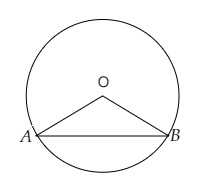# $A B$ is a chord of a circle with centre $O$ and radius $4 \mathrm{~cm} . A B$ is of length $4 \mathrm{~cm}$. Find the area of the sector of the circle formed by chord $A B$.

Given:

$A B$ is a chord of a circle with centre $O$ and radius $4 \mathrm{~cm} . A B$ is of length $4 \mathrm{~cm}$.

To do:

We have to find the area of the sector of the circle formed by chord $A B$.

Solution:The radius of the circle with centre $O= 4\ cm$

$\Rightarrow AO=BO=4\ cm$
Length of the chord $AB = 4\ cm$

This implies,

$\mathrm{AOB}$ is an equilateral triangle.

$\angle \mathrm{AOB}=60^{\circ}$

Area of the sector $\mathrm{AOB}=\pi r^{2} \times \frac{\theta}{360^{\circ}}$

$=\pi(4)^{2} \times \frac{60^{\circ}}{360^{\circ}} \mathrm{cm}^{2}$

$=16 \pi \times \frac{1}{6}$

$=\frac{8 \pi}{3} \mathrm{~cm}^{2}$

The area of the sector of the circle formed by chord $A B$ is $\frac{8 \pi}{3} \mathrm{~cm}^{2}$.

Updated on: 10-Oct-2022

38 Views